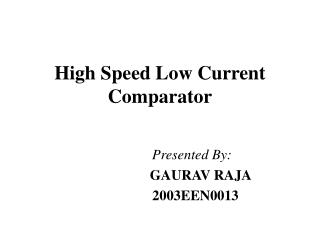DownloadDownload PresentationHigh Speed Low Current Comparator

# High Speed Low Current Comparator

Télécharger la présentation## High Speed Low Current Comparator

- - - - - - - - - - - - - - - - - - - - - - - - - - - E N D - - - - - - - - - - - - - - - - - - - - - - - - - - -
##### Presentation Transcript

1. High Speed Low CurrentComparator Presented By: GAURAV RAJA 2003EEN0013

2. Overview • Basic stages of current comparator • Simple current comparator • Proposed current comparator design • Simulation results • Conclusions • References

3. Basic stages of current comparator • Current to voltage conversion • Voltage amplification

4. Simple current comparator Current to voltage conversion Voltage amplification

5. Design specifications • Slew Rate >= 70 V/us • Load capacitor = 1 pF • Bias current = 70 uA • Input current switching between -100 nA to 100 nA • Reference current = 0 A Design is in such a way that it is not slew rate limited

6. Proposed current comparator 7.1u/1.2u 1u/.5u 0.7u/0.5u All transistors are assumed to be in saturation

7. Iin= 100 nA Delay time = 56 ns Simulation Results of simple current comparator Iin= 100 nA Delay time = 8 ns

8. Iin= 10 nA Iin= 100 nA Delay time = 21 ns Delay time = 12 ns Simulation Results of proposed current comparator Iin= 100 nA Delay time = 8 ns

9. Plot of delay time as a function of input current Simple CC Proposed CC

10. Simulation result for Vp=0.5 V

11. Proposed circuit

12. Simulation result for Vn=1.5 V

13. Conclusions • Delay time for 100 nA input current = 12 ns • Average power consumption = 0.53 mW • Delay time increases as the input current decreases. • Proposed circuit can work with 21 ns delay, even with 10 nA.

14. References • Hongchin Lin, Jie-Hau Huang, “A Simple High-Speed Low current comparator”, IEEE ISCAS-2000, May,1994. • P. Allen and D. Holberg, “CMOS Analog Circuit Design”, Oxford University Press, 2002.

15. Thank You